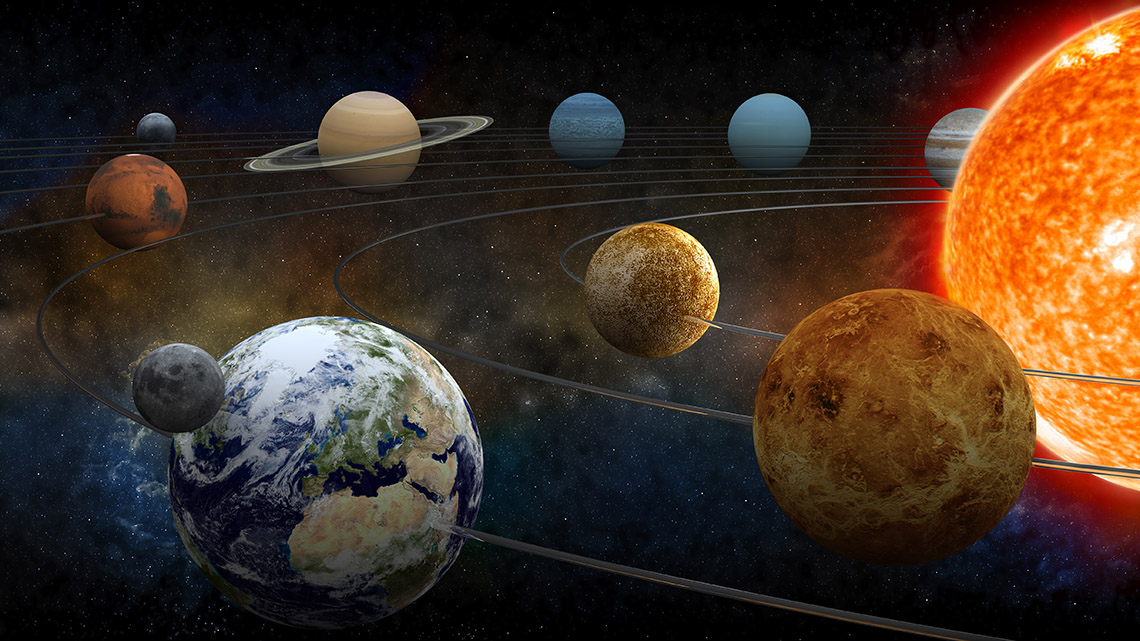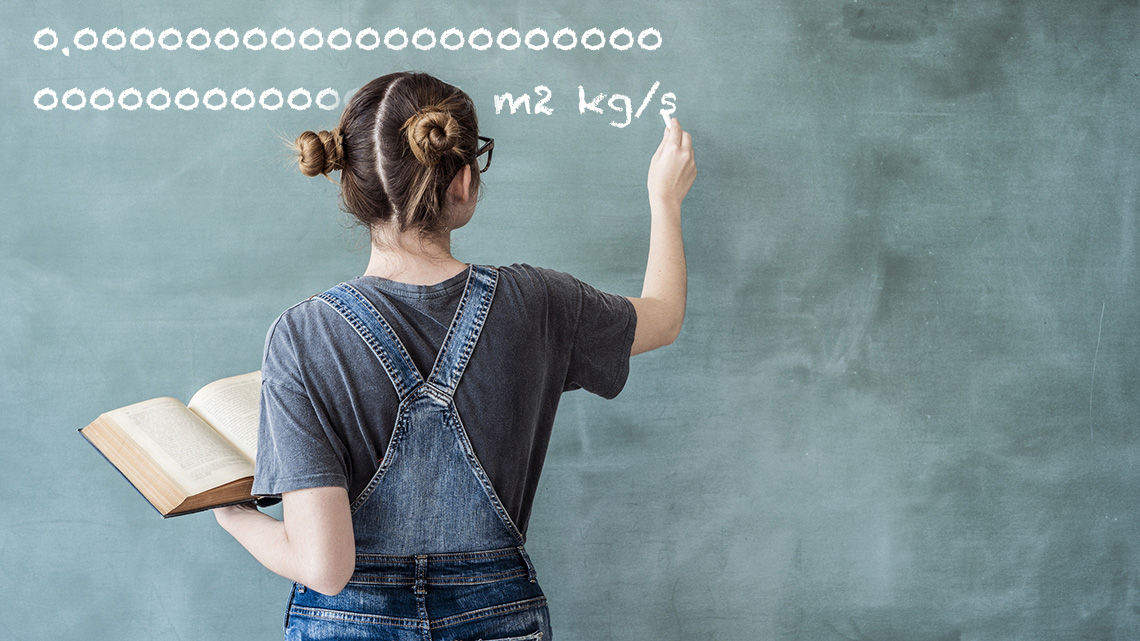# Minds On

## Scientific notation and astronomy

In this learning activity we will learn about scientific notation. Did you know that this important skill set is also used in the field of astronomy? Astronomers use scientific notation when measuring the distance between planets. Let’s get started to learn more about scientific notation!## Estimates of measurements

Think back to Grade 7 when you learned to use powers of ten to represent large numbers up to 1,000,000.

e.g., 100,000 = 105

We are now going to use those powers of 10 in even more complex ways!

Make estimates of the following measurements. When making an estimate there are no wrong answers, only answers that are closer than others.

Measurement Unit of Measurement: Estimate:
Mass of the Earth Kilograms (kg)
The distance from the Earth to the Sun Kilometres (km)
The length of ten years Seconds (s)
The width of the tip of a pin needle Centimetres (cm)
The number of grains of sand on Earth No unit
The number of cells in the human body No unit

The time it takes light to travel 1 metre

Seconds (s)

Come up with your own really big or really small thing to measure:

If you would like, you can complete this activity using TVO Mathify. You can also use your notebook or the following fillable and printable document.

Press the ‘TVO Mathify' button to access this interactive whiteboard and the ‘Activity’ button for your note-taking document. You will need a TVO Mathify login to access this resource.

TVO Mathify (Opens in new window) Activity (Open PDF in a new window)Student Success

### Think-Pair-Share

Think: Go back to your estimates.

• How did you come up with your estimate?
• Do you think any of your estimates might need changing?
• Could you write the written form of any of your estimates (e.g., one million, six thousandths, etc.)?

Note to teachers: See your teacher guide for collaboration tools, ideas and suggestions.

You probably had some estimates that were either really big numbers or really small numbers. They may have been something like this:

A Really Big Number: The Mass of the Earth in Kilograms A Really Small Number: The time it takes light to travel 1 metre
5,972,000,000,000,000,000,000,000 kg 0.000000003 seconds

Realistically, writing down these numbers whenever we might need them would take a lot of time and effort. Luckily, there is a faster way to write really big and really small numbers…

# Action

## Multiplying by powers of 10

### What is a power?Sometimes, we might need to multiply by the same number a bunch of times in a row. Rather than writing the long multiplication each time, we use powers or exponents.

Determine the powers for the following multiplication statements.

If you would like, you can complete this activity using TVO Mathify. You can also use your notebook or the following fillable and printable document.

Multiplication: Power:
10 × 10 102
10 × 10 × 10 103
10 × 10 × 10 × 10
10 × 10 × 10 × 10 × 10
10 × 10 × 10 × 10 × 10 × 10 × 10 × 10 × 10 × 10 × 10 × 10 × 10 × 10 × 10

Press the ‘TVO Mathify' button to access this interactive whiteboard and the ‘Activity’ button for your note-taking document. You will need a TVO Mathify login to access this resource.

TVO Mathify (Opens in new window) Activity (Open PDF in a new window)

If you would like, you can complete this activity using TVO Mathify. You can also use your notebook or the following fillable and printable document.

Multiplication: Power:
$\frac{1}{10}×\frac{1}{10}$ 10-2
$\frac{1}{10}×\frac{1}{10}×\frac{1}{10}$ 10-3
$\frac{1}{10}×\frac{1}{10}×\frac{1}{10}×\frac{1}{10}×\frac{1}{10}$
$\frac{1}{10}×\frac{1}{10}×\frac{1}{10}×\frac{1}{10}×\frac{1}{10}×\frac{1}{10}\phantom{\rule{0ex}{0ex}}×\frac{1}{10}×\frac{1}{10}$

Press the ‘TVO Mathify' button to access this interactive whiteboard and the ‘Activity’ button for your note-taking document. You will need a TVO Mathify login to access this resource.

TVO Mathify (Opens in new window) Activity (Open PDF in a new window)

### How you do multiply using powers of 10?Student Tips

### Multiplying using powers of 10

Multiplying a whole number and a decimal number by a positive power of ten can be visualized as shifting the digits to the left by one for each multiplication by 10.

Dividing a whole number and a decimal number by a power of 10 can be visualized as shifting the digits to the right by one for each division by 10.

For example, since 3.45 × 104 means the number 3.45 × 10 × 10 × 10 × 10 the digits "345" shift to the left four places to become 34,500.

For example, 3.45 ÷ 10 ÷ 10 ÷ 10, the digits “345” shift to the right three spaces to become 0.00345. And dividing by 10 is the same as multiplying by 0.1, thus 3.45 ÷ 10 ÷ 10 ÷ 10 is equal to 3.45 × 0.1 × 0.1 × 0.1 = 0.00345.

If you would like, you can complete this activity using TVO Mathify. You can also use your notebook or the following fillable and printable document.

Let's practice. Fill in the missing numbers in the following table.

Number: Multiply by 10 Multiply by $\frac{\mathbf{1}}{\mathbf{10}}$
0.76 7.6
0.05724
46

Press the ‘TVO Mathify' button to access this interactive whiteboard and the ‘Activity’ button for your note-taking document. You will need a TVO Mathify login to access this resource.

TVO Mathify (Opens in new window) Activity (Open PDF in a new window)

Recall that 10 x 10 = 100, 10 x 10 x 10 = 1000, etc. and that we can now use exponents instead of multiplication to show these bigger numbers.

Let's practice. Fill in the missing numbers in the following table.

If you would like, you can complete this activity using TVO Mathify. You can also use your notebook or the following fillable and printable document.

Number: Multiply by 10³ Multiply by 10-4 Multiply by 105
476.432 476,432 0.0476432 47,643,200
0.338
1.21
0.00034

Press the ‘TVO Mathify' button to access this interactive whiteboard and the ‘Activity’ button for your note-taking document. You will need a TVO Mathify login to access this resource.

TVO Mathify (Opens in new window) Activity (Open PDF in a new window)

## Scientific notationWhen we multiply by a power of 10 (for example: 10-4, 1012) the decimal moves either to the left or right when the numbers are smaller or larger.

We can use this to our advantage to write out long numbers in a shorter way called scientific notation. All we need is what power of 10 to multiply by and we find this by counting decimal places:So, the Mass of the Earth in kilograms is approximately: 5.972 × 10 24 which is a lot easier to write down than 5,972,000,000,000,000,000,000,000 kg!### Famous big and small numbers

The following table shows some famous big and small numbers. Why are they famous? These numbers are fundamental to our understanding of the Universe. From chemistry, to physics, to gravity and electricity. Without these numbers, we would be hundreds of years behind in technology as a society.

Your task will be to write each number in scientific notation. Remember to include the unit if a unit is provided.

If you would like, you can complete this activity using TVO Mathify. You can also use your notebook or the following fillable and printable document.

Famous Number: The Number: Scientific Notation:
Speed of light 300,000,000 m/s
Planck’s constant 0.000000000000000000000000
0000000006626 m² kg/s

Estimated number of atoms in the universe

100,000,000,000,000,000,000,000,000,000,
000,000,000,000,000,000,000,000,000,000,
000,000,000,000,000,000,000

Speed of sound

3.43 × 10²
Gravitational constant 0.00000000006673 m³ kg-1 s-2
Boltzmann’s constant 1.380650 × 1023 joule per kelvin

Press the ‘TVO Mathify' button to access this interactive whiteboard and the ‘Activity’ button for your note-taking document. You will need a TVO Mathify login to access this resource.

TVO Mathify (Opens in new window) Activity (Open PDF in a new window)

### Comparing numbers in scientific notation

Using the following table, write a number with a value between the two numbers surrounding it.. So, it is greater than the first number, and less than the second number.

• Complete the following chart in your notebook.
• You can write more than one answer.
• It might be helpful to draw or use a number line by plotting the first and second number, then finding one that would go in between.

If you would like, you can complete this activity using TVO Mathify. You can also use your notebook or the following fillable and printable document.

First Number: Middle Number: Second Number:
$5.32×{10}^{-4}$ $3.2×{10}^{-1}$
$1.2×{10}^{6}$ $4.2×{10}^{6}$
$6.543×{10}^{-10}$ $4.02×{10}^{-9}$
$1.001×{10}^{-5}$ $1.0001×{10}^{-4}$

Press the ‘TVO Mathify' button to access this interactive whiteboard and the ‘Activity’ button for your note-taking document. You will need a TVO Mathify login to access this resource.

TVO Mathify (Opens in new window) Activity (Open PDF in a new window)### Reflection

If possible, exchange your answers with a partner or with someone at home.

Make sure that all of the middle numbers are indeed in between the first and second numbers.

Consider the following questions. Be prepared to share your thinking in a discussion or in a recording.

• How could you find the number that is exactly in the middle? Develop a strategy and explain your thinking by finding it for at least one of the options.
• How many possible “middle numbers” are there in each question?

# Consolidation

## Scientific notation

### Part 1

Give at least 3 examples of numbers in scientific notation that are less than the following number, but as close to the number as you can make them:

5.32909 × 10-5

### Part 2

Next, give at least 3 examples of numbers in scientific notation that are greater than the number given and again, as close to the number as you can make them.

### Part 3How might you make your answers in parts 1 and 2 even closer to the given number? What is the closest possible number in each part?

### Reflection questions

• Why is it important to use scientific notation for very large or very small numbers?
• Write a number in scientific notation you think is interesting. Explain why you chose it.

## Reflection

As you read the following descriptions, select the one that best describes your current understanding of the learning in this activity. Press the corresponding button once you have made your choice.### I feel...

Now, expand on your ideas by recording your thoughts using a voice recorder, speech-to-text, or writing tool.

When you review your notes on this learning activity later, reflect on whether you would select a different description based on your further review of the material in this learning activity.

Press ‘Discover More’ to extend your skills.

Go back to the list of famous numbers from the Minds On section. Select one to research further. Try to answer the following questions for the number, keeping in mind it’s possible that not all these questions can be answered.

• What is the number used for?
• When was the number discovered?
• Who discovered it? Was there more than one person involved in its discovery?### Connect with a TVO Mathify tutor

Think of TVO Mathify as your own personalized math coach, here to support your learning at home. Press ‘TVO Mathify’ to connect with an Ontario Certified Teacher math tutor of your choice. You will need a TVO Mathify login to access this resource.

TVO Mathify (Opens in new window)